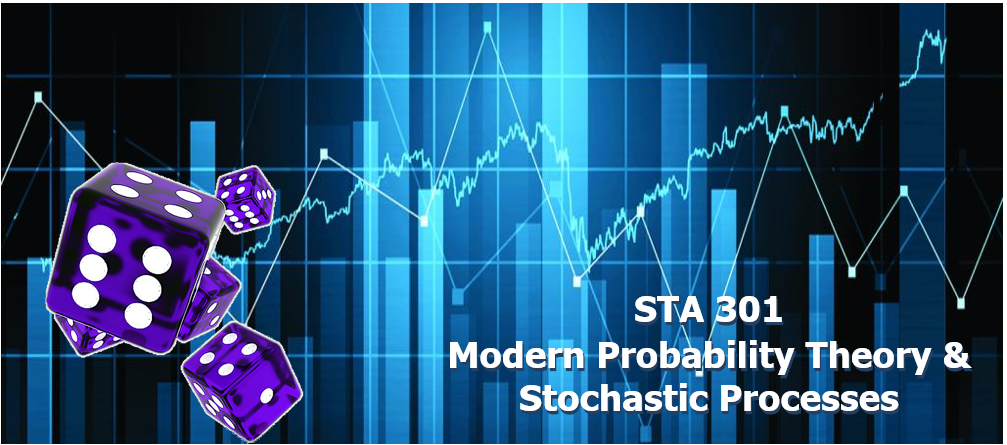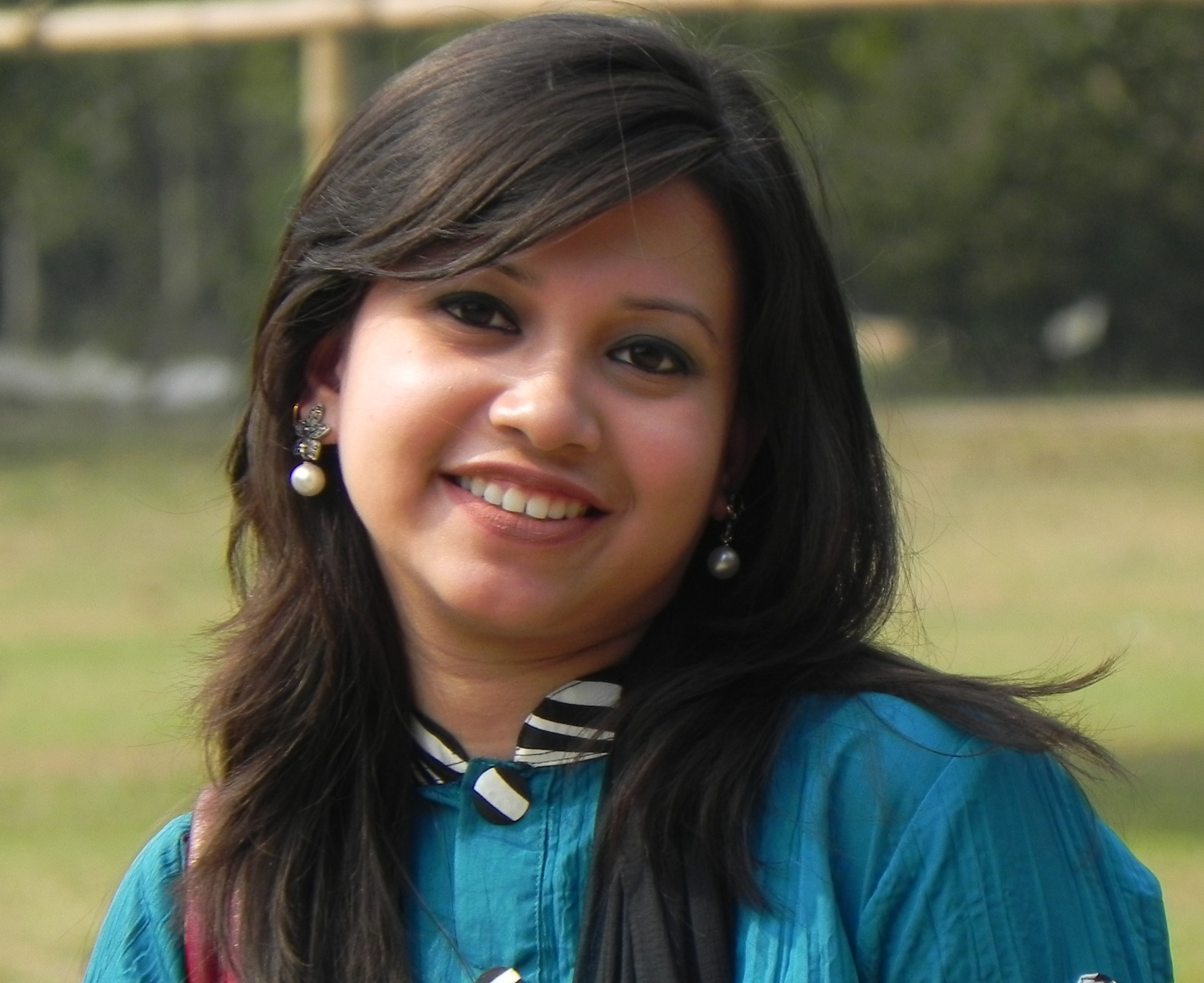# buX# Modern Probability Theory & Stochastic Processes (MNS) Enrollment is Closed

STA301: Modern Probability Theory and Stochastic Processes covers the mathematical theory of random variables and random processes for students who are planning to get involved and pursue high-end research-oriented engagement. The goal is to teach the theoretical concepts and techniques for solving problems that arise in practice. Beginning with the random variables, this course leads to the concept of the stochastic process and linear filtering of random processes.
Enrollment is Closed

STA301: Modern Probability Theory and Stochastic Processes covers two important parts for the students of science and engineering, one is advanced probability and another one is the stochastic process. This course will begin with a brief overview of modern probability and random distributions, the latter of which enables them to work with a stochastic process. Stochastic processes are ways of quantifying the dynamic relationships of sequences of random events. Stochastic models play an important role in elucidating many areas of sciences which include Markov chains, Poisson process, continuous-time Markov chain, and renewal process. It aims to bridge the gap between basic probability know-how and higher-level course in stochastic processes.

## Learning Outcome

Students who successfully complete this course should be able to:

CLO1: Derive the probability density function of random variables and use these techniques to generate data from various distributions.

CLO2: Explain the concepts of expectation and conditional expectation, and describe their properties.

CLO3: Analyse continuous and discrete-time random processes.

CLO4: Explain the concepts of stationary and wide-sense stationarity, and appreciate their significance.

CLO5: Apply the theory of stochastic processes to analyse linear systems.

CLO6: Apply the above knowledge to solve basic problems in filtering, prediction and smoothing.

## Faculty Members### Moumita Datta Gupta (MDG)

Course Coordinator and Lecturer (Statistics)